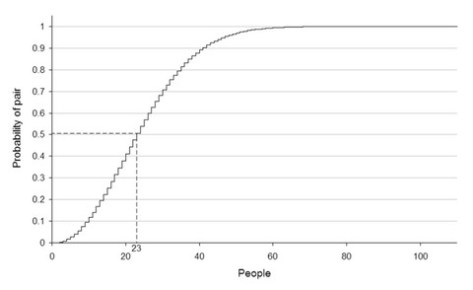# The Birthday Paradox: an interesting probability problem involving “statistically independent” events

During last week’s statistics tutorial, we discussed (among other things) the concept of statistical independence, and focused attention on some important implications of statistical independence for probability distributions such as the binomial and normal distributions.

Here, I’d like to call everyone’s attention to an interesting (non-finance) probability problem related to statistical independence. Specifically, consider the so-called “Birthday Paradox”. The Birthday Paradox pertains to the probability that in a set of randomly chosen people, some pair of them will have the same birthday. Counter-intuitively, in a group of 23 randomly chosen people, there is slightly more than a 50% probability that some pair of them will both have been born on the same day.

To compute the probability that two people in a group of n people have the same birthday, we disregard variations in the distribution, such as leap years, twins, seasonal or weekday variations, and assume that the 365 possible birthdays are equally likely. Thus, we assume that birth dates are statistically independent events. Consequently, the probability of two randomly chosen people not sharing the same birthday is 364/365. According to the combinatorial equation, the number of unique pairs in a group of n people is n!/2!(n-2)! = n(n-1)/2. Assuming a uniform distribution (i.e., that all dates are equally probable), this means that the probability that no pair in a group of n people shares the same birthday is equal to p(n) = (364/365)^[n(n-1)/2]. The event of at least two of the n persons having the same birthday is complementary to all n birthdays being different. Therefore, its probability is p’(n) = 1 – (364/365)^[n(n-1)/2].

Given these assumptions, suppose that we are interested in determining how many randomly chosen people are needed in order for there to be a 50% probability that at least two persons share the same birthday. In other words, we are interested in finding the value of n which causes p(n) to equal 0.50. Therefore, 0.50 = (364/365)^[n(n-1)/2]; taking natural logs of both sides and rearranging, we obtain (ln 0.50)/(ln 364/365) = n(n-1)/2. Solving for n, we obtain 505.304 = n(n -1); therefore, n is approximately equal to 23.

The following graph illustrates how the probability that a pair of people share the same birthday varies as the number of people in the sample increases:It is worthwhile noting that real-life birthday distributions are not uniform since not all dates are equally likely. For example, in the northern hemisphere, many children are born in the summer, especially during the months of August and September. In the United States, many children are conceived around the holidays of Christmas and New Year’s Day. Also, because hospitals rarely schedule C-sections and induced labor on the weekend, more Americans are born on Mondays and Tuesdays than on weekends; where many of the people share a birth year (e.g., a class in a school), this creates a tendency toward particular dates. Both of these factors tend to increase the chance of identical birth dates, since a denser subset has more possible pairs (in the extreme case when everyone was born on three days of the week, there would obviously be many identical birthdays!).

Note that since 77 students are enrolled in two sections of Finance 4335 this semester, this implies that the probability that two Fall 2019 Finance 4335 students share the same birthday is p’(77) = 1 – (364/365)^[77(76)/2] = 99.97%, although given footnote 1’s caveats, it’s likely that there may be several shared birthday pairs.